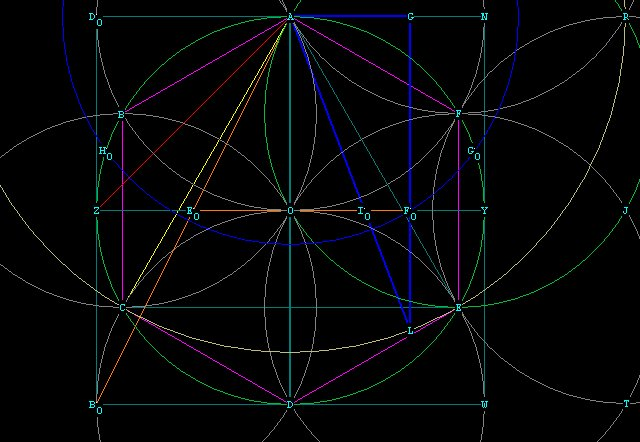CONTINUING THE EXPLORATION OF THE CONVERSION TRIANGLE

Now we continue with the analysis of the Ø  to \/¯3 conversion triangle.
Notice that in Figure 1, the line OFo intersects the hypotenuse and the long leg of the triangle at
Io and Fo respectively.
The line OFo divides  not ony the long side GL, but also the hypotenuse AL, in Mean and Extreme Ratio. Let's show this:

GFo = OA = radius  = 1.
FoL  = 1 / Ø.
Consider the triangle LFoIo. It is similar, by angle-angle-angle, to the larger triangle AGL.
Therefore, GL is to AL as FoL is to IoL.
We can then write:
FoL / IoL  =  Ø / \/¯3 , and  (1 / Ø)  / IoL =   Ø / \/¯3,  IoL =  \/¯3 / Ø².
Then AIo = AL - IoL  =  \/¯3  - (\/¯3 / Ø²)
= [( \/¯3Ø² - \/¯3) /  Ø²]  =  [\/¯3( 1 - Ø²) /  Ø²]
= \/¯3Ø /  Ø²   =  \/¯3 / Ø.
AIo / IoL = (\/¯3 / Ø)  /  (\/¯3 / Ø²)   =  Ø.
Therefore AL is divided in Mean and Extreme Ratio at Io.Figure 1  --- the Ø  to \/¯3 conversion traingle AGL

With this same relationship we can also write:
FoL / IoFo = GL / AG  =   Ø / (1 / Ø) = Ø².
IoFo = FoL / Ø²  =  (1 / Ø) /  Ø²  =  1 / Ø³.

OIo = OFo - IoFo  =  (1 / Ø) - 1 / Ø³  =  1 / Ø².

Therefore the line AL, representing the  \/¯3, divides the line OFo, representing the  \/¯5,
in Mean and Extreme Ratio at Io!

This example also serves to demonstrate, geometrically, the inverse of Ø², and Ø³.
It is easy to add a known distance to another known distance, but it is another thing altogether to demonstrate the accurate division into a known quantity. For instance, one of the impossible things to do with a 2 dimensional compass is to trisect a known line segment.
Here, we have demonstrated the division into an irrational quantity based upon the \/¯5, and have done it with the \/¯3!!

Not only that, but we have also demonstrated the 1 / Ø²  and the 1 / Ø³ triangles and their relationship to .the \/¯3 geometrically.
The 1 / Ø² triangle is triangle AOIo.
The 1 / Ø³ triangle is triangle IoFoL.
The 1 / Ø² triangle we have shown is a 1, 1 / Ø² , \/¯3 / Ø triangle.
The 1 / Ø³ triangle we have shown is a 1 / Ø,  1 / Ø³,  \/¯3 / Ø² triangle.
These relationships resolve  mathematically to the same equation as the  conversion triangle, and this is shown geometrically by the fact that the triangles are similar.
I believe it is important, when doing geometry, to establish a real basis for the symbolism of mathematics. Without physical demonstrability, mathematics becomes irrelevant. Fun, but irrelevant.

There is now no question of the relationship between  Ø and the \/¯3.

Special characters:
\/¯ ­ ° ¹ ² ³ × ½ ¼ Ø  \/¯(Ø² + 1)